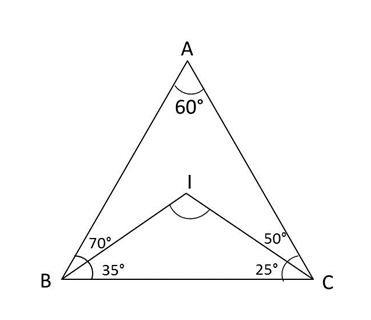# CAT Quantitative Aptitude Questions | Geometry Questions for CAT - Triangles

###### CAT Questions | CAT Geometry Questions | Triangles

The question is from the topic triangles. It discusses about incenter of a triangle. CAT Geometry questions are heavily tested in CAT exam. Make sure you master Geometry problems. Two angles of a triangle is given, we need to find out the angle made by incenter and vertices.

Question 20: Triangle ABC has angles A = 60° and B = 70°. The incenter of this triangle is at I. Find angle BIC.

1. 90°
2. 130°
3. 80°
4. 120°

## Best CAT Coaching in Chennai

#### CAT Coaching in Chennai - CAT 2022Limited Seats Available - Register Now!

##### Method of solving this CAT Question from Triangles: What is an incenter? Clue - It has something to do with angle bisectors.A + B + C = 180 in a triangle
Hence C = 180 – 130 = 50°
Incenter is the meeting point of angle bisectors. BIC will form a triangle.
In this triangle B = 35, C = 25
Hence BIC or I = 180 – (35 + 25) = 120°

The question is "Find angle BIC."

##### Hence, the answer is 120°.

Choice D is the correct answer.

###### CAT Coaching in ChennaiCAT 2023

Classroom Batches Starting Now! @Gopalapuram

###### Best CAT Coaching in Chennai Introductory offer of 5000/-

Attend a Demo Class

## CAT Preparation Online | CAT Geometry questions Videos On YouTube

#### Other useful sources for Geometry Question | Geometry Triangles Circles Quadrilaterals Sample Questions

##### Where is 2IIM located?

2IIM Online CAT Coaching
A Fermat Education Initiative,
58/16, Indira Gandhi Street,
Kaveri Rangan Nagar, Saligramam, Chennai 600 093

##### How to reach 2IIM?

Phone: (91) 44 4505 8484
Mobile: (91) 99626 48484
WhatsApp: WhatsApp Now
Email: prep@2iim.com